﻿

### Generalization of certain well-known inequalities for rational functions

#### Abstract

Let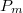$P_m$ be a class of all polynomials of degree at most m and let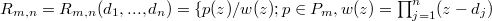$R_{m,n}=R_{m,n}(d_{1}, ..., d_{n})=\{p(z)/w(z);p\in P_{m}, w(z)=\prod_{j={1}}^n(z-d_{j})~$where$~ \vert d_{j}\vert\ >1, j=1, ..., n$ and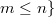$m\leq n\}$ denote the class of rational functions. It is proved that if the rational function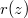$r(z)$ having all its zeros in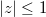$\vert z\vert\leq1$, then for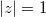$\vert z\vert=1$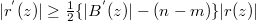$\vert r^{'}(z)\vert\geq \frac{1}{2}\{\vert B^{'}(z)\vert-(n-m)\} \vert r(z)\vert$.

The main purpose of this paper is to improve the above inequality for rational functions$r(z)$ having all its zeros in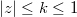$\vert z\vert\leq k\leq1$ with$t$-fold zeros at the origin and some other related inequalities. The obtained results sharpen some well-known estimates for the derivative and polar derivative of polynomials.

DOI Code: 10.1285/i15900932v40n1p1

Keywords: Rational functions; Polynomials; Polar derivative; Inequalities; Poles; Restricted Zeros

Full Text: PDF